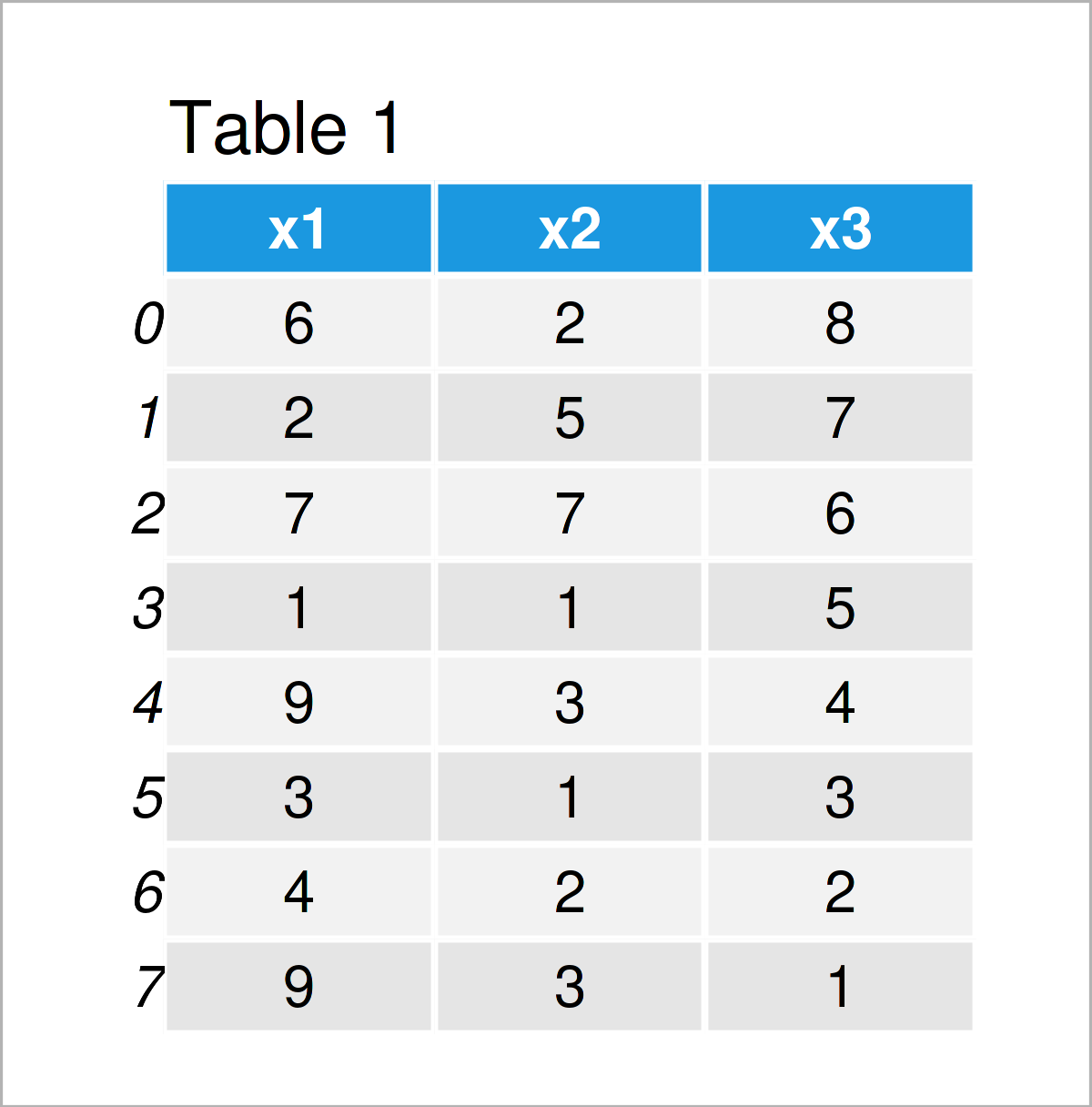# Sum of Columns & Rows of pandas DataFrame in Python (2 Examples)

In this tutorial you’ll learn how to calculate the sums of columns and rows of a pandas DataFrame in the Python programming language.

The content is structured as follows:

Let’s dive right into the examples…

## Example Data & Libraries

In order to use the functions of the pandas library, we first have to load pandas:

`import pandas as pd                                   # Load pandas`

Furthermore, have a look at the following example data:

```data = pd.DataFrame({'x1':[6, 2, 7, 1, 9, 3, 4, 9],  # Create example DataFrame
'x2':[2, 5, 7, 1, 3, 1, 2, 3],
'x3':range(8, 0, - 1)})
print(data)                                          # Print example DataFrame```Have a look at the previous table. It shows that our example data consists of eight rows and three columns called “x1”, “x2”, and “x3”.

## Example 1: Calculate Sum of Each Column in pandas DataFrame

The following Python code explains how to get the column sums of all numeric variables of a pandas DataFrame in Python.

For this, we can use the sum function as shown below:

```print(data.sum())                                    # Get column sums
# x1    41
# x2    24
# x3    36
# dtype: int64```

Have a look at the previous Python console output: It shows the result of adding all values in a column. The sum of all values in the column x1 is 41, the sum of the variable x2 is 24, and the sum of column x3 is 36.

## Example 2: Calculate Sum of Each Row in pandas DataFrame

In Example 2, I’ll explain how to compute the row sums of all rows of a pandas DataFrame.

Similar to Example 1, we can use the sum function for this task. However, in order to return the row sums instead of the column sums, we have to specify the axis argument within the sum function to be equal to 1:

```print(data.sum(axis = 1))                            # Get row sums
# 0    16
# 1    14
# 2    20
# 3     7
# 4    16
# 5     7
# 6     8
# 7    13
# dtype: int64```

The previous console output shows the sum of each row in our example data set.

## Video, Further Resources & Summary

Would you like to know more about the sum of columns and rows of a pandas DataFrame? Then I can recommend having a look at the following video on my YouTube channel. In the video, I show the Python programming code of this article and explain the syntax in more detail:

Please accept YouTube cookies to play this video. By accepting you will be accessing content from YouTube, a service provided by an external third party.If you accept this notice, your choice will be saved and the page will refresh.

As an additional resource, I recommend having a look at the following video on the Chart Explorers YouTube channel. The speaker is explaining the topics of this article in the video:

Please accept YouTube cookies to play this video. By accepting you will be accessing content from YouTube, a service provided by an external third party.If you accept this notice, your choice will be saved and the page will refresh.

In addition, you might want to read the related Python programming articles on this homepage.

Summary: This article has explained how to compute the sums of columns and rows of a pandas DataFrame in the Python programming language. Please tell me about it in the comments, if you have any additional comments or questions.

Subscribe to the Statistics Globe Newsletter